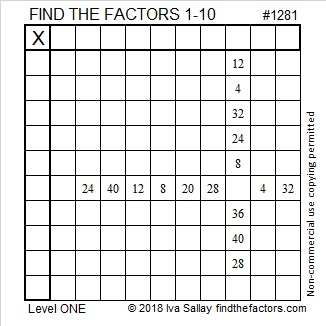# 1281 and Level 1

Can you write the numbers from 1 to 10 in the top row and the first column so that the given clues will make this puzzle work like a multiplication table? That’s how you solve the puzzle!Print the puzzles or type the solution in this excel file: 10-factors-1281-1288

Now I’ll write a little bit about the number 1281:

• 1281 is a composite number.
• Prime factorization: 1281 = 3 × 7 × 61
• The exponents in the prime factorization are 1, 1, and 1. Adding one to each and multiplying we get (1 + 1)(1 + 1)(1 + 1) = 2 × 2 × 2 = 8. Therefore 1281 has exactly 8 factors.
• Factors of 1281: 1, 3, 7, 21, 61, 183, 427, 1281
• Factor pairs: 1281 = 1 × 1281, 3 × 427, 7 × 183, or 21 × 61
• 1281 has no square factors that allow its square root to be simplified. √1281 ≈ 35.791061281 is also the sum of consecutive prime numbers in two different ways:
167 + 173 + 179 + 181 + 191 + 193 + 197 = 1281
241 + 251 + 257 + 263 + 269 = 1281

1281 is the hypotenuse of a Pythagorean triple:
231-1260-1281 which is 21 times (11-60-61)

This site uses Akismet to reduce spam. Learn how your comment data is processed.